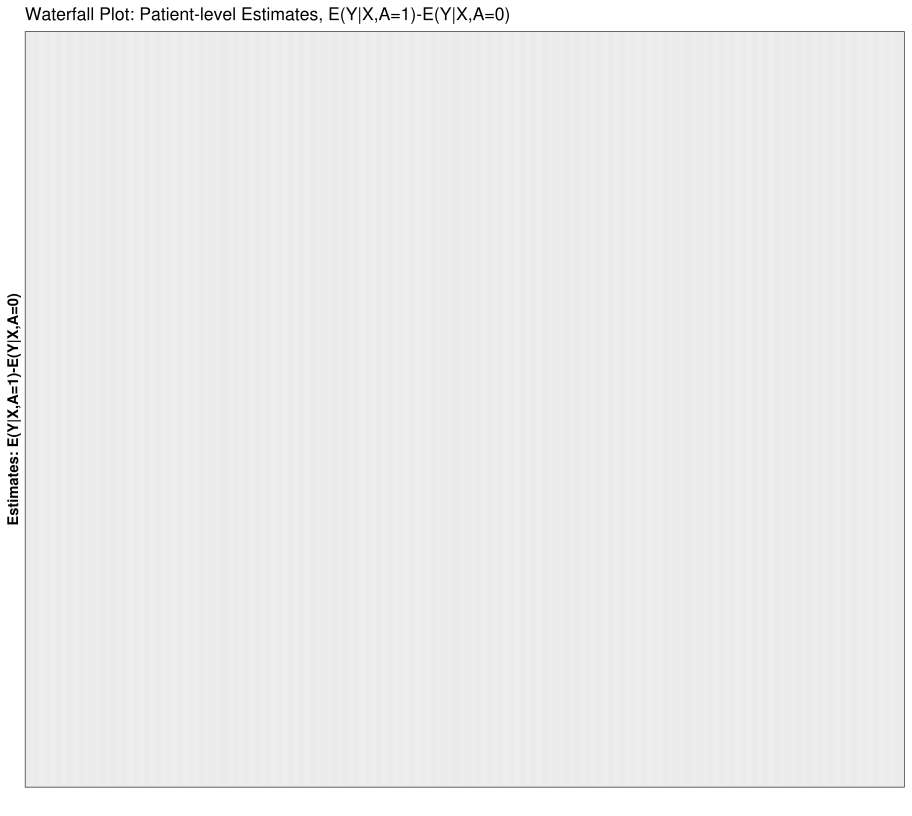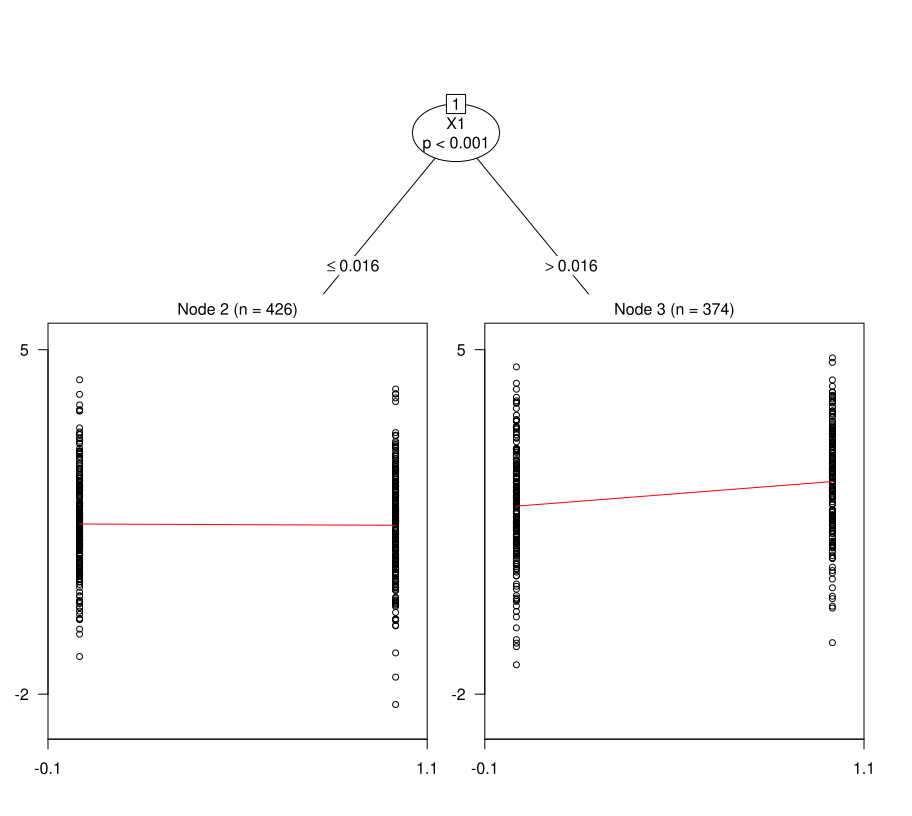User Specific Models

One advantage of the “StratifiedMedicine”" package is the flexibility to input user-created functions/models. This facilitates faster testing and experimentation. First, let’s simulate the continuous data again.

library(StratifiedMedicine)
dat_ctns = generate_subgrp_data(family="gaussian")
Y = dat_ctns\$Y
X = dat_ctns\$X # 50 covariates, 46 are noise variables, X1 and X2 are truly predictive
A = dat_ctns\$A # binary treatment, 1:1 randomized

Next, before we illustrate how to implement user-specific models in PRISM, let’s highlight the key outputs at each step.

Key Outputs by Model
Model Required.Outputs Description
filter filter.vars Variables that pass filter
ple list(mod,pred.fun) Model fit(s) and prediction function
submod list(mod,pred.fun) Model fit(s) and prediction function
param param.dat Treatment Effect Estimates

For the filter model (“filter_train”), the only required output is a vector of variable names that pass the filter (for example: covariates with non-zero coefficients in elastic net model). For the patient-level estimate model (“ple_train”) and subgroup model (“submod_train”), the required outputs are the model fit(s) and an associated prediction function. The prediction function can also be swapped with pre-computed predictions (details below). Lastly, for parameter estimation (param), the only required output is “param.dat”, a data-frame containing point-estimates/SEs/CIs.

Filter Model (filter)

The template filter function is:

filter_template = function(Y, A, X, ...){
# Step 1: Fit Filter Model #
mod <- # model call
# Step 2: Extract variables that pass the filter #
filter.vars <- # depends on mod fit
# Return model fit and filtered variables #
res = list(mod=mod, filter.vars=filter.vars)
return( res )
}

Note that the filter uses the observed data (Y,A,X), which are required inputs, and outputs an object called “filter.vars.” This needs to contain the variable names of the variables that pass the filtering step. For example, consider the lasso:

filter_lasso = function(Y, A, X, lambda="lambda.min", family="gaussian", ...){
require(glmnet)
## Model matrix X matrix #
X = model.matrix(~. -1, data = X )

##### Elastic Net ##
set.seed(6134)
if (family=="survival") { family = "cox"  }
mod <- cv.glmnet(x = X, y = Y, nlambda = 100, alpha=1, family=family)

### Extract filtered variable based on lambda ###
VI <- coef(mod, s = lambda)[,1]
VI = VI[-1]
filter.vars = names(VI[VI!=0])
return( list(filter.vars=filter.vars) )
}

An option to change lambda, which can change which variables remain after filtering (lambda.min keeps more, lambda.1se keeps less), while not required, is also included. This can then be adjusted through the “hyper” and “filter.hyper” arguments in “filter_train” and “PRISM” respectively.

Patient-Level Estimates (“ple_train”)

The template ple function is:

ple_template <- function(Y, A, X, ...){
# Step 1: Fit PLE Model #
# for example: Estimate E(Y|A=1,X), E(Y|A=0,X), E(Y|A=1,X)-E(Y|A=0,X)
mod <- # ple model call
# mod = list(mod0=mod0, mod1=mod1) # If multiple fitted models, combine into list
# Step 2: Predictions
# Option 1: Create a Prediction Function #
pred.fun <- function(mod, X, ...){
mu_hat <- # data-frame of predictions
return(mu_hat)
}
# Option 2: Directly Output Predictions (here, we still use pred.fun) #
mu_train <- pred.fun(mod, X)
mu_test <- pred.fun(mod, Xtest)

# Return model fits and pred.fun (or just mu_train/mu_test) #
res <- list(mod=mod, pred.fun=pred.fun, mu_train=mu_train, mu_test=mu_test)
return( res )
}

For “ple_train”, the only required arguments are the observed data (Y, X). The only required outputs are mod (fitted models(s)) and a prediction function or pre-computed predictions in the training/test set (mu_train, mu_test). If the training/test set predictions are provided, this will be used instead of the prediction function. However, certain features in “StratifiedMedicine”, such as PDP plots (“plot_dependence”), cannot be utilized without providing a prediction funcion. In the example below, we set up a simple wraper for random forest based predictions. This base-learner can then be combined with the default meta-learners (meta=“T-learner”, “X-learner”, “S-learner”) to obtain predictions for specific exposures or treatment differences.

ple_ranger_mtry = function(Y, X, mtry=5, ...){
require(ranger)
train =  data.frame(Y=Y, X)
mod <- ranger(Y ~ ., data = train, seed=1, mtry = mtry)
mod = list(mod=mod)
pred.fun <- function(mod, X, ...){
mu_hat <- predict(mod\$mod, X)\$predictions
mu_hat <- data.frame(mu_hat)
return(mu_hat)
}
res = list(mod=mod, pred.fun=pred.fun)
return(res)
}

Subgroup Identification (“submod_train”)

The template submod function is:

submod_template <- function(Y, A, X, Xtest, mu_train, ...){
# Step 1: Fit subgroup model #
mod <- # model call
# Step 2: Predictions #
# Option 1: Create Prediction Function #
pred.fun <- function(mod, X=NULL, ...){
Subgrps <- # Predict subgroup assignment
return( list(Subgrps=Subgrps) )
}
# Option 2: Output Subgroups for train/test (here we use pred.fun)
Subgrps.train = pred.fun(mod, X)
Subgrps.test = pred.fun(mod, X)
#Return fit and pred.fun (or just Subgrps.train/Subgrps.test)
res <- list(mod=mod, pred.fun=pred.fun, Subgrps.train=Subgrps.train,
Subgrps.test=Subgrps.test)
return(res)
}

For the “submod” model, the only required arguments are the observed data (Y,A,X). “mu_train” (based on “ple_train” predictions) can also be passed through. The only required outputs are mod (fitted models(s)) and a prediction function or pre-computed subgroup predictions in the training/test set (Subgrps.train, Subgrps.test). In the example below, consider a modified version of “submod_lmtree” where we search for predictive effects only. By default, “submod_lmtree” searches for prognostic and/or predictive effects.

submod_lmtree_pred = function(Y, A, X, mu_train, ...){
require(partykit)
## Fit Model ##
mod <- lmtree(Y~A | ., data = X, parm=2) ##parm=2 focuses on treatment interaction #
pred.fun <- function(mod, X=NULL, type="subgrp"){
Subgrps <- NULL
Subgrps <- as.numeric( predict(mod, type="node", newdata = X) )
return( list(Subgrps=Subgrps) )
}
## Return Results ##
return(list(mod=mod, pred.fun=pred.fun))
}

Parameter Estimation (“param_est”)

The template param function is:

param_template <- function(Y, A, X, mu_hat, alpha,...){
# Key Outputs: Subgroup specific and overall parameter estimates
mod <- # Call parameter model #
# Extract estimates/variability and combine #
param.dat <- data.frame(n=n, estimand="mu_1-mu_0",
est=est, SE=SE, LCL=LCL, UCL=UCL, pval=pval)
return(param.dat)
}

For the parameter model, key arguments are (Y, A) (observed outcome/treatment) and alpha (nominal type I error for CIs). Other inputs can include mu_hat (ple predictions) and the covariate space (X) if needed for parameter estimation. The only required output is “param.dat”, which contains parameter estimates/variability metrics. For all PRISM functionality to work, param.dat should contain column names of “est” (parameter estimate), “SE” (standard error), and “LCL”/“UCL” (lower and upper confidence limits). It is recommended to include an “estimand” column for labeling purpose. In the example below, M-estimation models are fit for each subgroup and overall.

### Robust linear Regression: E(Y|A=1) - E(Y|A=0) ###
param_rlm = function(Y, A, alpha, ...){
require(MASS)
indata = data.frame(Y=Y,A=A)
rlm.mod = tryCatch( rlm(Y ~ A , data=indata),
error = function(e) "param error" )
n = dim(indata)
est = summary(rlm.mod)\$coefficients[2,1]
SE = summary(rlm.mod)\$coefficients[2,2]
LCL =  est-qt(1-alpha/2, n-1)*SE
UCL =  est+qt(1-alpha/2, n-1)*SE
pval = 2*pt(-abs(est/SE), df=n-1)
param.dat <- data.frame(N= n, estimand = "mu_1-mu_0",
est=est, SE=SE, LCL=LCL, UCL=UCL, pval=pval)
return(param.dat)
}

Putting it All Together

Finally, let’s input these user-specific functions into each step along with combining the components with PRISM:

step1 <- filter_train(Y, A, X, filter="filter_lasso")
X.star <- X[,colnames(X) %in% step1\$filter.vars]
step2 <- ple_train(Y, A, X.star, ple = "ple_ranger_mtry")
plot_ple(step2)
#> Warning: Removed 800 rows containing missing values (position_stack).step3 <- submod_train(Y, A, X.star, submod = "submod_lmtree_pred")
plot(step3\$fit\$mod)step4 <- param_est(Y, A, X.star, Subgrps=step3\$Subgrps.train, param="param_rlm")
step4
#>   Subgrps   N  estimand          est         SE         LCL       UCL
#> 1    ovrl 800 mu_1-mu_0  0.234728163 0.07708495  0.08341524 0.3860411
#> 2       2 426 mu_1-mu_0 -0.004165125 0.10352507 -0.20765001 0.1993198
#> 3       3 374 mu_1-mu_0  0.506836561 0.11525208  0.28021130 0.7334618
#>           pval alpha
#> 1 2.402725e-03  0.05
#> 2 9.679263e-01  0.05
#> 3 1.429483e-05  0.05
# PRISM #
res_user1 = PRISM(Y=Y, A=A, X=X, family="gaussian", filter="filter_lasso",
ple = "ple_ranger_mtry", submod = "submod_lmtree_pred",
param="param_rlm")
plot_ple(res_user1)
#> Warning: Removed 800 rows containing missing values (position_stack).